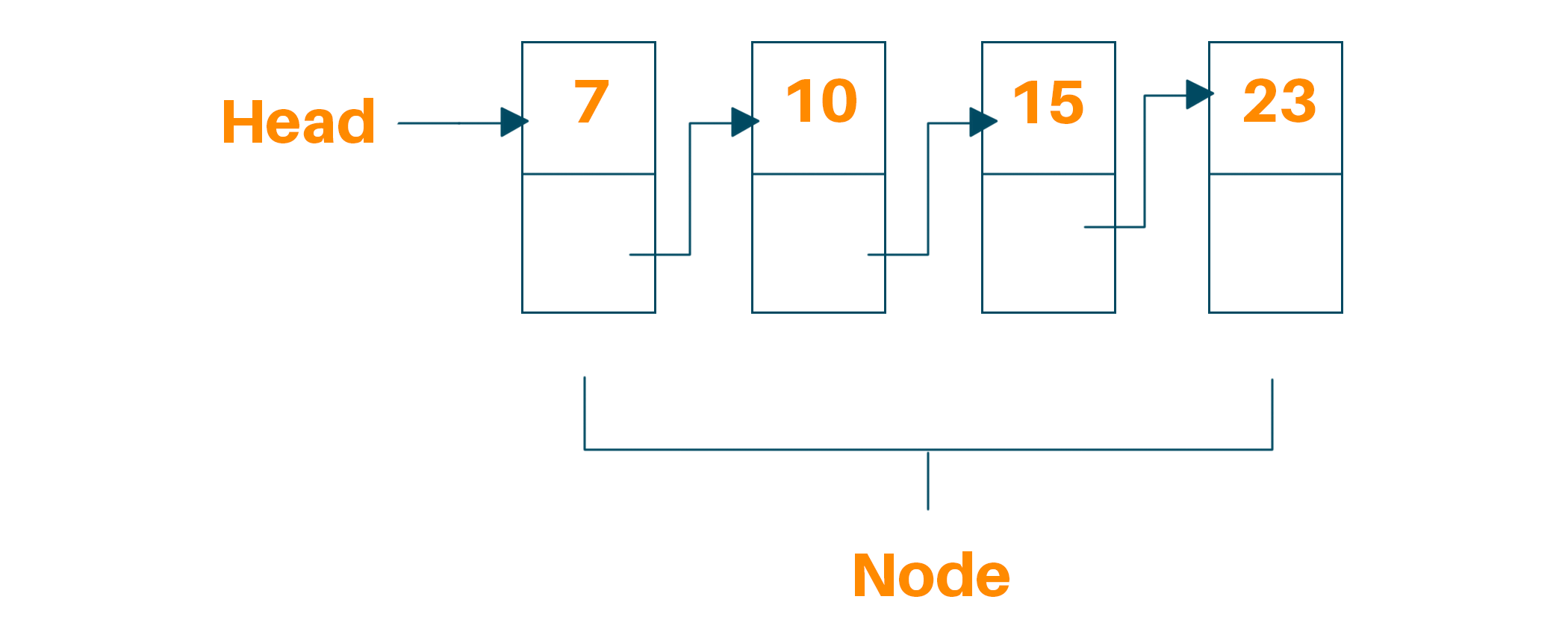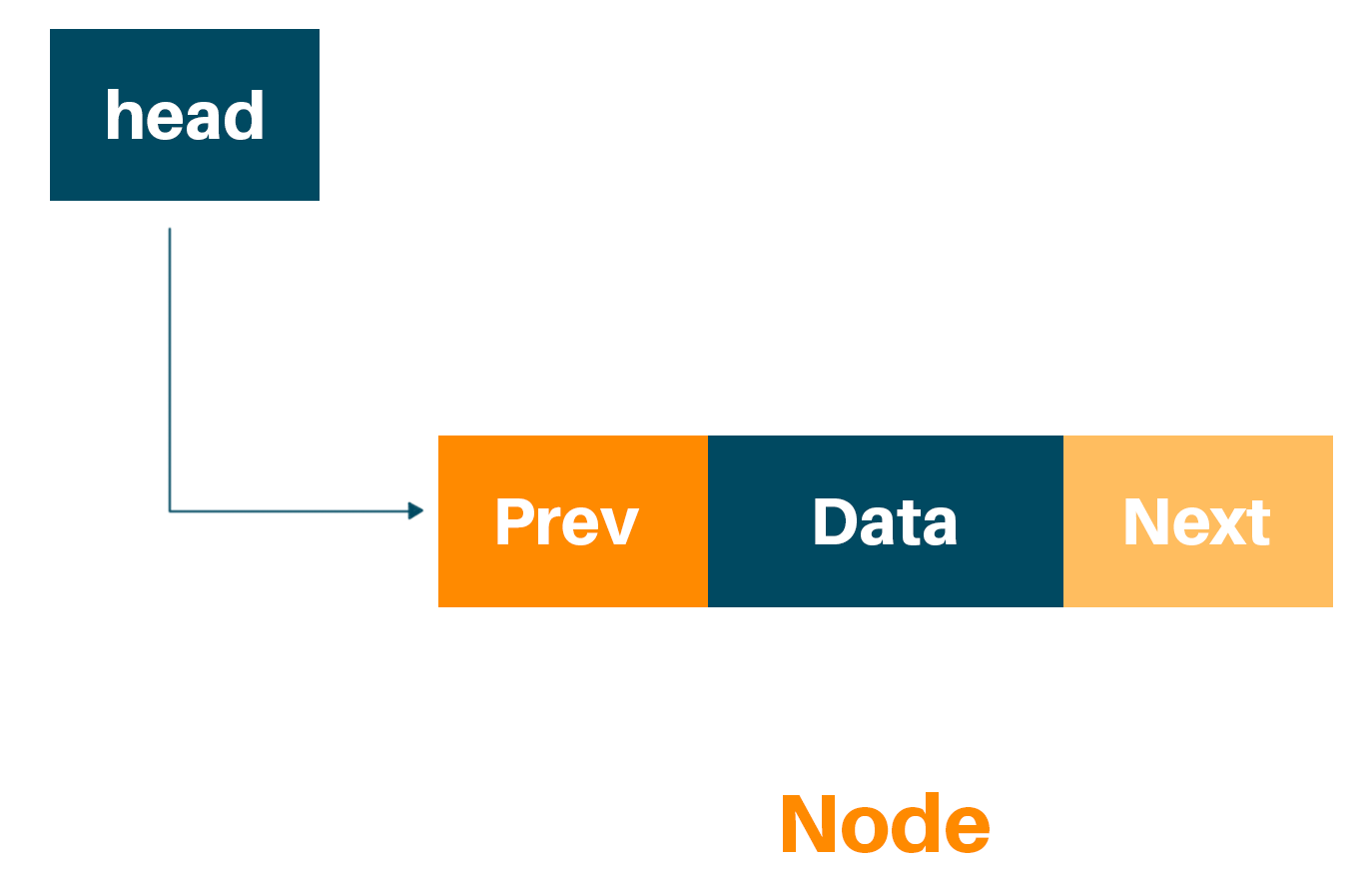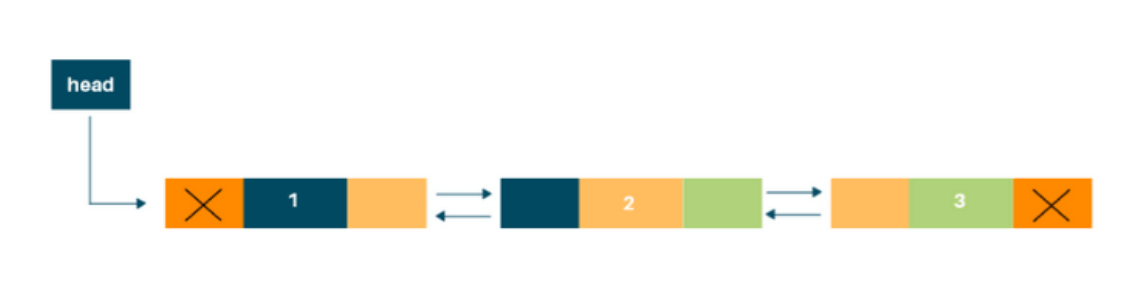# Doubly Linked List (Python Code with Example)

Ctrl+Z, can you even survive a day without these keys? Well, Apparently not, undo and redo features are one of the used functionalities in computer engineering. But have you ever thought about how is it even possible? I happen to have an answer this time! Undo and Redo functionality is one of the most important applications of a doubly linked list. So what exactly is a doubly linked list? Before that let’s have a quick glance at Linked List:

Linked list is a linear data structure in which each node is an object. Different from general Arrays, the Linked list data structure does not have a contiguous memory structure. Every element is connected to the subsequent node through a pointer.

Every element present in the Linked list is known as Node. Every node contains a key or data element with an extra pointer pointing to the next element in the list. The initial pointer in the  Linked list is called Head. It is necessary to state that “Head” pointer is not another node but a pointer to the first element of the Linked list. If the Linked list are empty value of Head will be null.### Basic implementation of Linked List in Python is:

```class Node:
def __init__(self, dataval=None):
self.dataval = dataval
self.nextvnextal = None

def __init__(self):

e2 = Node(90)
e3 = Node(90)
# Link first Node to second node

# Link second Node to third node
e2.nextval = e3
```

## What is Doubly Linked List ?

It is easier to implement Singly Linked list or Linked list,whereas it is pretty difficult to traverse that in reverse, to overcome this we can utilise Doubly LinkedList, where every node takes an additional pointer to point the former node to the element in addition to the pointer for the next node.

A doubly linked list has more efficient iteration, particularly if you need to ever iterate in reverse and more efficient deletion of particular nodes.

We can conclude that a doubly linked list is a complex type of linked list where a node contains a pointer to the previous as well as the next node in the sequence. Therefore, in a doubly-linked list, a node consists of three components: node data, pointer to the next node in node(next pointer), pointer to the former node (previous pointer).### Example

For example, consider a doubly linked list containing four nodes having, four numbers from 1 to 4 in their data part, illustrated in the following image## Step-by-Step Implementation of Doubly Linked List in Python

We will implement doubly linked list using some concepts of object oriented programming in python. We will first create a class for creating a node in a basic linked list, with three attributes: the data, previous pointer and next pointer. The code looks like this:

```class Node:
def __init__(self, data):
self.item = data
self.next = None
self.prev = None
```

The item variable will store the actual element of the node. The next stores the address to the next node, while prev stores the address to the previous node in the doubly linked list.

In the next step, we will create a doublyLinkedList class, that contains different functions to insert, delete and display elements of doubly linked list.

```class doublyLinkedList:
def __init__(self):
self.start_node = None
```

1. Inserting Items to Empty List
2. Inserting Items at the End
3. Deleting Elements from the Start
4. Deleting Elements from the End

### Inserting Items to Empty List

The most permissive way to insert an element in a doubly linked list is to insert the element in the empty list. The following piece of code enters an element at the origin of the doubly linked list:

```    def InsertToEmptyList(self, data):
if self.start_node is None:
new_node = Node(data)
self.start_node = new_node
else:
print("The list is empty")
```

### Inserting Items at the End

Inserting an element at the end of the doubly linked list is slightly alike to inserting an element at the start of the list. Before entering the elements, we need to verify and check if the doubly linked list is empty. If the list is empty then we can easily use the function InsertToEmptyList() method to insert the element to the list. If the list already has added element, we iterate through the list till the address to the next node reaches NULL. When the next node address becomes NULL, it denotes that the current node is the last node.

The previous reference for the new node is set to the last node, and the next address for the last node is assigned to the newly inserted node. The function for inserting an item at the last node is as follows:

```    # Insert element at the end
def InsertToEnd(self, data):
# Check if the list is empty
if self.start_node is None:
new_node = Node(data)
self.start_node = new_node
return
n = self.start_node
# Iterate till the next reaches NULL
while n.next is not None:
n = n.next
new_node = Node(data)
n.next = new_node
new_node.prev = n
```

### Deleting Elements from the Start

The most natural way to delete an element from a doubly linked list is deleting from the start of the list. In order to achieve this, we will assign the value of the start node to the next node and then assign the previous address of the start node to NULL. But before doing this, we need to check two things. First, we need to check if the list is empty. And then we have to verify if the list has only one node or not. If the list has only one element then we can easily assign the start node to NULL. The following code can be utilised to delete node from the start of the doubly linked list.

```    # Delete the elements from the start
def DeleteAtStart(self):
if self.start_node is None:
print("The Linked list is empty, no element to delete")
return
if self.start_node.next is None:
self.start_node = None
return
self.start_node = self.start_node.next
self.start_prev = None;
```

### Deleting Elements from the End

To remove the element from the end, we have to again verify if the list is empty or if the list has a single element. If the list has a single element, we will assign the start node to NULL. If the doubly linked list has more than one element, we traverse through the list until the last node reaches NULL. Once we reach the last node, we assign the next address of the node previous to the last node, to NULL which actually deletes the last node. The following function can be used to delete the element from the end of the list.

```    # Delete the elements from the end
def delete_at_end(self):
# Check if the List is empty
if self.start_node is None:
print("The Linked list is empty, no element to delete")
return
if self.start_node.next is None:
self.start_node = None
return
n = self.start_node
while n.next is not None:
n = n.next
n.prev.next = None
```

Display the elements in the doubly linked list, while iterating through each element.

```    # Traversing and Displaying each element of the list
def Display(self):
if self.start_node is None:
print("The list is empty")
return
else:
n = self.start_node
while n is not None:
print("Element is: ", n.item)
n = n.next
print("\n")
```

## Complete python code of Doubly Linked List

```# Initialise the Node
class Node:
def __init__(self, data):
self.item = data
self.next = None
self.prev = None
# Class for doubly Linked List
def __init__(self):
self.start_node = None
# Insert Element to Empty list
def InsertToEmptyList(self, data):
if self.start_node is None:
new_node = Node(data)
self.start_node = new_node
else:
print("The list is empty")
# Insert element at the end
def InsertToEnd(self, data):
# Check if the list is empty
if self.start_node is None:
new_node = Node(data)
self.start_node = new_node
return
n = self.start_node
# Iterate till the next reaches NULL
while n.next is not None:
n = n.next
new_node = Node(data)
n.next = new_node
new_node.prev = n
# Delete the elements from the start
def DeleteAtStart(self):
if self.start_node is None:
print("The Linked list is empty, no element to delete")
return
if self.start_node.next is None:
self.start_node = None
return
self.start_node = self.start_node.next
self.start_prev = None;
# Delete the elements from the end
def delete_at_end(self):
# Check if the List is empty
if self.start_node is None:
print("The Linked list is empty, no element to delete")
return
if self.start_node.next is None:
self.start_node = None
return
n = self.start_node
while n.next is not None:
n = n.next
n.prev.next = None
# Traversing and Displaying each element of the list
def Display(self):
if self.start_node is None:
print("The list is empty")
return
else:
n = self.start_node
while n is not None:
print("Element is: ", n.item)
n = n.next
print("\n")
# Create a new Doubly Linked List
# Insert the element to empty list
# Insert the element at the end
# Display Data
# Delete elements from start
# Delete elements from end
# Display Data
```

## Applications

• A music player that has next and previous buttons, like the concept of doubly linked list you’ll have functionality to go back and the next element. .
• Undo Redo operations that we discussed at the beginning of the article.
• The browser cache functionality in common web browsers like Chrome, Microsoft edge, which allows us to move front and back within pages is also a great application of a doubly linked list.
• LRU cache, also Most Recently Used is also an instance of DLL.
• A deck of cards in a game is a perfect example of the applicability of DLL. It is additionally utilized to store the state of the game during play.

## Conclusions